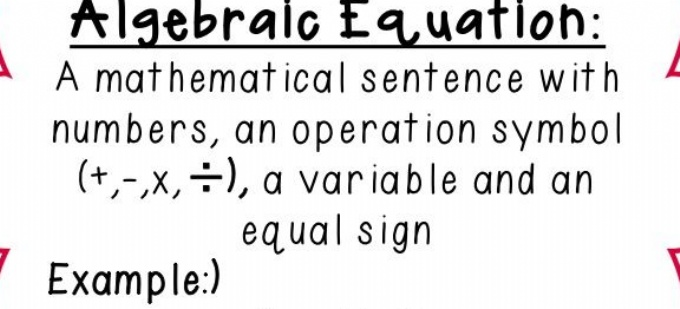# Algebraic EquationsThis week we have continued with our Algebra topic.

Today we have been learning how to create algebraic equations. This is the first step to being able to solve algebraic equations. An equation is a number sentence that has at least one letter (a variable), numbers, an operation sign (x, +, - or /) and an equal sign.

Here are some of the equations we wrote today...

r - 6 = 16

a + 5 = 25

(i x 6 ) + 3 = 75

d x 6 = 24

We realised that we can use any letters to signify the number we don't know but that using o was not a good idea as it looks too much like a zero!

We also learnt that n x 6 can also be written as 6n.

Tomorrow we move on to solving algebraic equations...